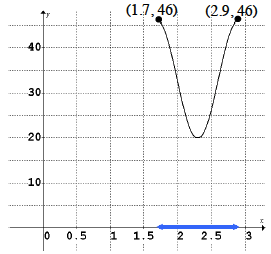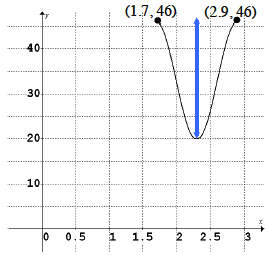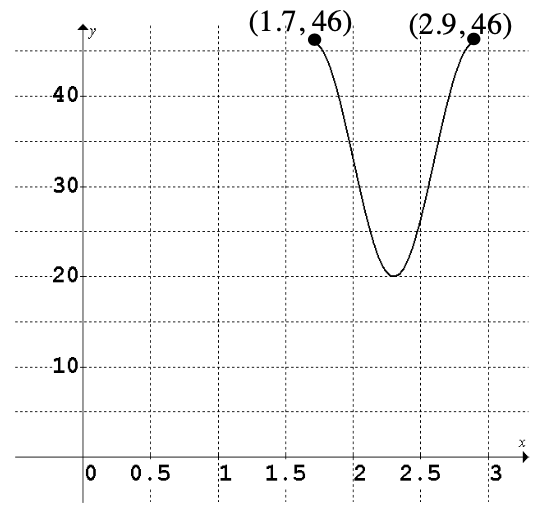### Home > PC > Chapter 7 > Lesson 7.1.2 > Problem7-28

7-28.

Lauren is playing with a yo-yo and keeping track of its height over time. She has plotted some key points on the graph at right. The $y$-values are in inches and the $x$-values are in seconds. Complete the following problems based on the graph.

1. How long does it take to complete one cycle?2. How long is the string?3. Find an equation that will model the height of the yo-yo over time.

Find:
1. Amplitude
2. Vertical Shift
3. Horizontal Shift
4. $b$ (frequency)

Note: part (a) is the period and $pb = 2π$
4. Find the first two times when the yo-yo was exactly $30$ inches above the ground.
Find where the function crosses $y = 30$.